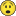# QlikView App Dev

Discussion Board for collaboration related to QlikView App Development.

cancel
Showing results for
Did you mean:Creator III

## Rangeavg bug with zero values?

Hi I have a pivot table where I'm using rangeavg +  agg to calculate the last 3 months average for current month. It works like a charm when there are no zeros in any of the months, but when there are, the result is 0 even when there's data for the other 2 months.

Do you know the solution to this?

1 Solution

Accepted SolutionsMVP

See if one of these work for you

RangeAvg(After(TOTAL Sum({<MONTH1=,MONTH=,MONTH_NAME=,YEAR=,QUARTER=,Q=, SUBJECT={'DELIVER'}>} LN_COUNT),0,3))

or

RangeAvg(After(TOTAL Sum({<MONTH1=,MONTH=,MONTH_NAME=,YEAR=,QUARTER=,Q=>} IF(SUBJECT='DELIVER', LN_COUNT,0)),0,3))

8 RepliesMVP

May be do RangeSum() and divide by 3?Creator III
Author

I tried that, but rangesum is returning 0 as well.....

sum(aggr(Rangesum(above(sum({<MONTH1=,MONTH=,MONTH_NAME=,YEAR=,QUARTER=,Q=>}IF(SUBJECT='DELIVER', LN_COUNT,0)),0,3)),CUSTOMER_CHANNEL_GROUP,CUST_RTM_GROUP,SEGMENT,PODI_GROUPED,PLANNING_STRATEGIC_REGION_CD,MONTH))/3MVP

Would you be able to share an example where it isn't working?Creator III
Author

Here's the dashboard I have, in the container is the tab "Order", column "Den" (this is the one that calcualtes the 3 months avg)

The data for the average is the one in the tab "Delivery" column "Den"

I have 1 big fact table with a "Subject" table.

I actually noticed that there's no "DELIVER" data in Sept for MCA, this is causing the 0....

Any Idea how to fix it?MVP

Where do I see MCA? I am hard time finding it in the sample. Apologize if it is right thereCreator III
Author

No worries, I didn't make myself clear. Expand the last dimension column to see it... I just ran a dummy example with an inline load..... so it won't calculate unless  at least one expression has data for Sep... in this particular neither Num nor Den has data for Sep.... I will have to generate dummy data for each record at its lowest level I guessany idea how to easily solve this?MVP

See if one of these work for you

RangeAvg(After(TOTAL Sum({<MONTH1=,MONTH=,MONTH_NAME=,YEAR=,QUARTER=,Q=, SUBJECT={'DELIVER'}>} LN_COUNT),0,3))

or

RangeAvg(After(TOTAL Sum({<MONTH1=,MONTH=,MONTH_NAME=,YEAR=,QUARTER=,Q=>} IF(SUBJECT='DELIVER', LN_COUNT,0)),0,3))Creator III
Author

Thank you very much Sir. I didn't know this could be done without using aggrCommunity Browser